# Land area

The land area of Asia and Africa are in a 3: 2 ratio, the European and African are is 1:3. What are the proportions of Asia, Africa, and Europe?

Result

x = (Correct answer is: 9:6:2)### Step-by-step explanation: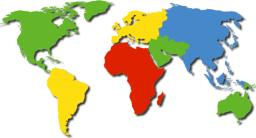Did you find an error or inaccuracy? Feel free to write us. Thank you!

Showing 1 comment:Math student
Drag the cards below to create an expression that is equal to \dfrac72
2
7

start fraction, 7, divided by, 2, end fraction.Tips to related online calculators
Need help to calculate sum, simplify or multiply fractions? Try our fraction calculator.
Check out our ratio calculator.

## Related math problems and questions:

• Land area 2A land area was divided among the three heirs in the ratio 5:2:4. If the largest share was 20 hectares of land, what is the total area of land? Please show your solution and what kind of proportion is this please
• Sign of a expressionWhat is the sign of expression: minus 18 start fraction 9 divided by 17 end fraction plus left parenthesis minus 18 start fraction 9 divided by 17 end fraction right parenthesis?
• Two brothersThe two brothers were to be divided according to the will of land at an area of 1ha 86a 30m2 in a ratio of 5:4. How many will everyone get?
• Composite ratioJakub, Aneta, and Lenka divided 1342 USD in the ratio 5/2: 3/10: 1/4. How much did Lenka take?
• Fraction expressionWhich expression is equivalent to : minus 9 minus left parenthesis minus 4 start fraction 1 divided by 3 end fraction right parenthesis
• Three 43Three brothers inherited a cash amount of 62,000, and they divided it among themselves in the ratio of 5:4:1. How much more is the largest share than the smallest share?
• The land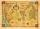The land in the shape of a square has 9 ha. How big a page will the land have at the scale of 1: 5000?
• In theIn the national park, the ratio of the wooded area to grassland is 4: 1. The total area is 385km2. What area is wooded?
• Brass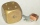Brass is an alloy of copper and zinc in a ratio of 3: 2. How many grams does a component that required 270 g of copper weigh?
• Fraction + eqSolve following simple equation with fractions: -5/6(8+5b) = 75 + 5/3b
• BuildingAt the building, we divided 240 boards into two piles in a 5: 3 ratio. How many were fewer boards in the lower pile?
• Ratio of sides 2The ratio of the side lengths of one square to another is 1:2. Find the ratio of the area of the two squares.
• Over fractionSolve equation: p over 5 equals fraction numerator 7 p over denominator 6 end fraction plus 5
• Sprinter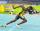Sprinter runs the relay 4 x 400 m to the handover at a speed of 42 km/h. A second runner is at the start of the handover area 20 m long and runs when it is the first sprinter at a distance of 10 m. Calculate the speed at which the second runner must run t
• Squares ratioThe first square has a side length of a = 6 cm. The second square has a circumference of 6 dm. Calculate the proportions of the perimeters and the proportions of the contents of these squares? (Write the ratio in the basic form). (Perimeter = 4 * a, conte
• ExpressionIf it is true that (l + 15 w)/(w) = 6, then the value of the expression (13 l)/(12 w) is:
• Ratio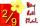Write the ratio with other numbers so that the value is the same: 2: 9